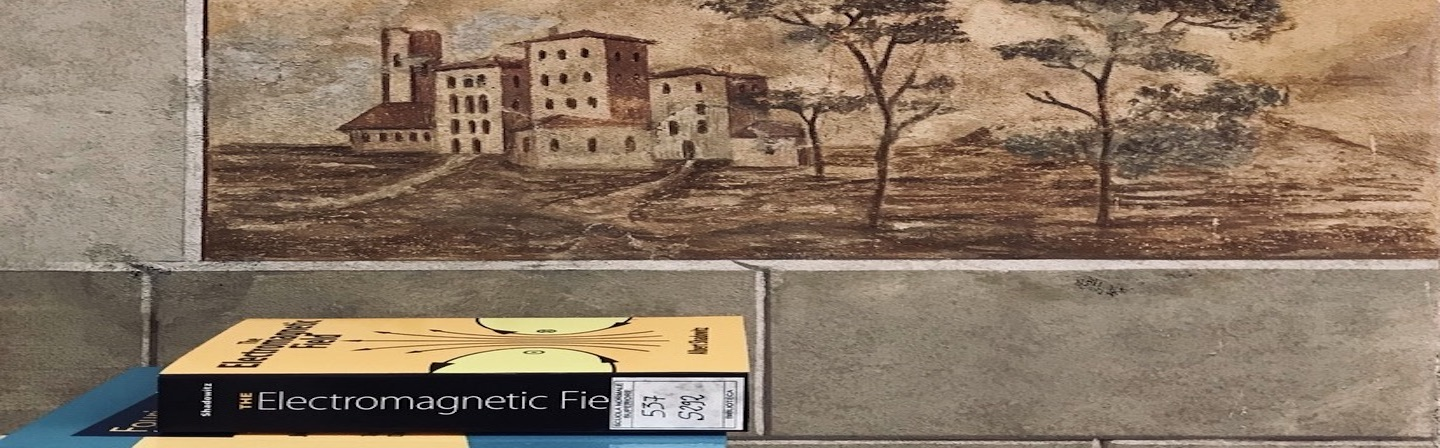# Sciences

#### Coverage by subjects

The monograph collection is divided into the following main sections: Mathematical logic, Linear Algebra, Topology, Analysis, Geometry, Astronomy, Physics, Chemistry, Biology, Applied sciences (sections of Medicine and Engineering), Computer Science.

The main topics explored by the collection are: Mathematical logic, Linear algebra, Number theory, Topological algebra, Analysis, Calculus and differential equations, partial differential equations, Algebraic geometry, Differential geometry, Numerical analysis , Probability, Mathematical finance, Astrophysics, Theoretical physics, Theory of relativity, Quantum statistical mechanics, Field theories, Mathematical physics, Physics of matter and solid state physics, Classical mechanics and Thermodynamics, Electrodynamics, Nuclear and particle physics, Physical chemistry, Quantum chemistry, Biophysics, Cellular and molecular biology, Biochemistry, Neurosciences, Nanotechnologies, Automatic control engineering, Computer science.

#### Authors' libraries relevant to Sciences and the main topics represented

Luigi Bianchi, for differential geometry, analysis, number theory
Enrico Betti, for analysis, algebra, mathematical physics, works by famous authors of physics and mathematics (18th - 19th centuries)
Cesare Finzi, per l’algebra, la geodesia, opere di classici della fisica e della matematica (sec. XVIII-XIX)
Giancarlo Wick, for algebra, geodesics, works by famous authors of physics and mathematics (18th - 19th centuries)
Clifford Truesdell, for mathematical physics, fluid mechanics, works by famous authors of physics and mathematics (16th - 20th centuries)

#### Periodicals

The periodical literature for this study area includes an open shelf print collection and a collection of e-journals. Most of the titles subscribed to by the Library are consultable online.
A print collection of periodicals, in exchange with other institutions, is displayed on open shelves at the Canonica site (the last two years' issues).
The previous years' issues of the the printed periodicals are located at the book deposit, on closed shelves.

#### Location

The collection of open shelf monographs is located in Pisa, on the ground floor of the Canonica site.
In the classification scheme there are listed the main thematic sections of the collection, placed according to the Dewey Decimal Classification (23 ed.), and highlighted on the shelf through the corresponding subject.
Housed at the Capitano site, in the Sala di Storia della Scienza ‘Clifford Truesdell’ (the ‘Clifford Truesdell’ History of Science Room), is the historic-scientific collection, on closed shelves, with editions of the nineteenth century and the first decades of the twentieth century, in which are merged the collections of the Scuola Normale and parts of the authors' libraries, for which please refer to the specific sheet in the section Personal collections and Authors’ libraries.

#### New acquisitions

The list of new acquisition is published in the SEARCH catalogue and gives an account of the titles acquired in the last 2 months.

Multidisciplinary databases

Chemistry databases

Physics databases

Mathematics and Computer Science databases

Biological sciences databases